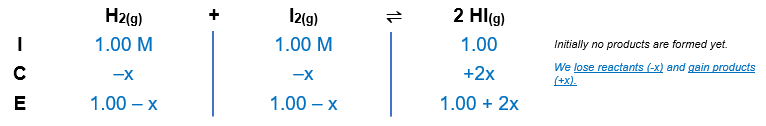# Problem: At a particular temperature, K = 1.00 x 102 for the reactionH2(g) + I2(g) ⇌ 2HI(g)In an experiment, 1.00 mole of H2, 1.00 mole of I2, and 1.00 mole of HI are introduced into a 1.00-L container. Calculate the concentrations of all species when equilibrium is reached.

###### FREE Expert Solution

H2(g) + I2(g) ⇌ 2 HI(g)          K = 1.00 x 102

Step 1: Calculate the concentrations of the reactants and products.

Recall molarity

[H2] = 1.00 M

[I2] = 1.00 M

[HI] = 1.00 M

Step 2: Calculate the reaction quotient.

$\overline{){\mathbf{Q}}{\mathbf{=}}\frac{\mathbf{products}}{\mathbf{reactants}}}$

$\overline{){\mathbf{Q}}{\mathbf{=}}\frac{\mathbf{products}}{\mathbf{reactants}}}$

$\mathbf{Q}\mathbf{=}\frac{{\mathbf{\left[}\mathbf{HI}\mathbf{\right]}}^{\mathbf{2}}}{\mathbf{\left[}{\mathbf{H}}_{\mathbf{2}}\mathbf{\right]}\mathbf{\left[}{\mathbf{I}}_{\mathbf{2}}\mathbf{\right]}}\phantom{\rule{0ex}{0ex}}\mathbf{Q}\mathbf{=}\frac{{\mathbf{\left(}\mathbf{1}\mathbf{.}\mathbf{00}\mathbf{\right)}}^{\mathbf{2}}}{\mathbf{\left(}\mathbf{1}\mathbf{.}\mathbf{00}\mathbf{\right)}\mathbf{\left(}\mathbf{1}\mathbf{.}\mathbf{00}\mathbf{\right)}}$

Q = 1.00

Q < K → the reaction shifts in the forward direction to reach equilibrium

Step 3: Construct an ICE chart for the equilibrium reaction.Step 4: Calculate the change (x) in the reaction using the equilibrium constant.

83% (95 ratings)###### Problem Details

At a particular temperature, K = 1.00 x 102 for the reaction

H2(g) + I2(g) ⇌ 2HI(g)

In an experiment, 1.00 mole of H2, 1.00 mole of I2, and 1.00 mole of HI are introduced into a 1.00-L container. Calculate the concentrations of all species when equilibrium is reached.